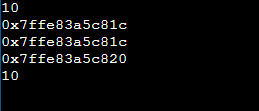# Pointers in C++

## Pointers in C++ in Depth with Examples:

Hello, Guys welcome back to another and very important article in C++ Basics Called Pointers with Examples. Most of the programmers found this topic difficult and confusing. So, in this article, I will try to explain most of the concepts in layman terms rather than more technical terms. Please read our previous article where we discussed Arrays in C++. Pointers are the types of variables in C++. These are also in C language, so a similar thing is there in C++.

##### What is a Pointer?

If I directly give you the definition of pointer trust me you won’t get it. So, let’s directly take an example and discuss what exactly a pointer is in C++?

int x=10; Here x is a variable that holds the value 10. But in memory 10 is stored as shown in the below image.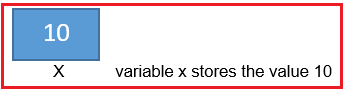In the above example, x is a normal variable. The following is an example of a pointer variable.

int *ptr=&x;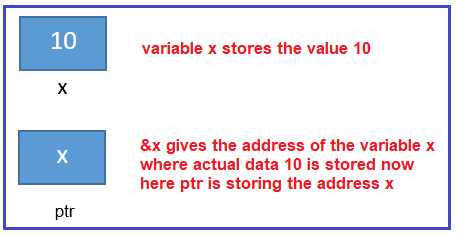So, if you ask me to define a pointer, then I would define the pointer as follows.

Pointers are variables that store the address of another variable where the value resides. That means the pointer is a variable that is used for storing the address of data. So, we can categorize variables of two types,

1. Data Variable

Data Variable is used for storing data whereas the Address Variable is used for storing address.

int x = 10;

Here we have an integer type variable x having the value 10. So x is for storing data and hence it is a data variable. When we declare the variables, they will occupy some memory depending on the size of the data type. For explanation, we are taking the size of int data type is 2 bytes. For better understanding, please have a look at the below image.Let us assume that the addresses are 200 and 201. Actually, a modern compiler will take 4 bytes. But if we take four bytes in the explanation then it will be too long. So that’s why we are taking 2 bytes integer.

##### How to declare an Address variable in C++?

int p; Here we have declared a variable with the name p and here p means it is a data variable. Now how to make it an address variable?

int *p; Here p should be prefixed with *. Once it is prefixed with *, then it becomes an address variable. The address variables in C++ cannot store the data but they can store the address of the data. As the address variables are variables, they also occupy memory. In our example, the address variable p is occupying 2 bytes. For better understanding, please have a look at the below image.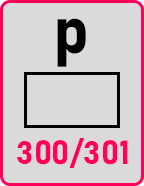Let us say that addresses are 300 and 301.

p = &x; Now here we have assigned &x to p. So, what is the address of x? 200, So that 200 will be stored in the address variable p. For better understanding, please have a look at the below image.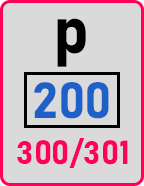So here it will be 200. It will be pointing to x. It is having the address of x. It’s not having data but having the address of ‘x’. Now let us see the syntax.

int *p; This is the declaration of address variable.
*p = &x; This is the initialization of a pointer variable. Let us write some printing statements and see the output.
cout << x;
Output: 10
x is containing 10, so 10 will be displayed.

cout << &x;
Output: 200
&x means the address of x that is 200. So, 200 will be displayed.

cout << p;
Output: 200
It will be showing the contents of p that is 200 will be displayed.

cout << &p;
Output: 300
Here 300 will be displayed.

Now the final and the important one that is
cout << *p;
Output: 10
This will be displaying the data where p is pointing. And p is pointing at 200 at that place 10 is stored. So, this is called dereferencing or accessing the data where p is pointing. Below are the 3 important statements:
Declaration: int *p;
Initialization: p = &x;
dereferencing: cout << *p;

So, what we have learned here is how to declare a pointer and how to use a pointer, how to dereference a pointer.

##### Declaration of Pointer Variables in C++:

Pointer declaration is similar to the variable declaration but to distinguish pointer variable from normal variable we need to use ‘*’ asterisk while declaring pointer variable.
General Syntax: <Datatype> *var_name;
Examples:
int *ptr_int; //pointer-to-integer
char *ptr_char; //pointer-to-character
bool *ptr_bool; //pointer-to-boolean

##### Initialization of Pointers in C++:

We can initialize the pointer at the time of declaration and we can initialize the pointer after declaration. I will show you how to do it.
Example:
int x=10;
int *ptr_int = &x; //initialization at the time of declaration of pointer
int x=10;
int *ptr_int;
ptr_int=&x; //initialization after declaration.

Note: While initialization of pointer after the declaration, we should not use * asterisk along with pointer_variable_name. Commonly for normal variables we just do declaration and thereafter initialization same thing we did for pointers and even you don’t see any difference in that except the use of asterisk ‘*’. But for pointers, there is another important thing we usually do i.e. dereference the pointer.

##### What is pointer dereferencing and how do pointer dereferencing work in C++?

Again, if directly give the definition it is not easy to understand so let’s directly dive into an example
Example:
int x=10;
int *ptr=&x;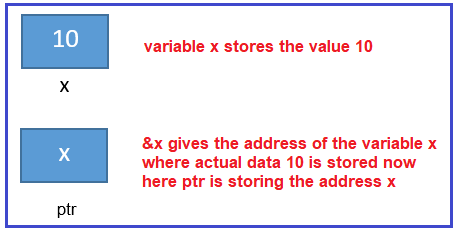The same example I have taken which I used previously. Now I want to access a value 10 from ptr how I can access it? Can you Think????

Yes, I know ptr knows the address of x means currently ptr is referring to x. If I somehow think is there a way to get value from the address means if I know how to defer it then I could get the value 10. Let’s say x has the address 100. ptr has a value 100 which is the address of the variable x where 10 is stored;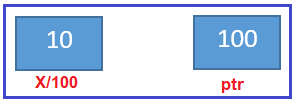If I print &x then I will get the value 100
If I print ptr then I will get the value it is holding means 100
If I print just &ptr then I will get the address ptr as it is also variable let’s say it is to address it 110.

##### But now I need to print value 10 from ptr. is it possible?

Yes, it is possible through pointer dereferencing. To derefer pointer, we need to use the asterisk ‘*’. This means if I print *ptr then I will get the value 10.

##### How????

‘*’ associated with ptr tells the compiler to fetch the value associated with the stored address. Here stored address in ptr is 100 and the use of * tells the compiler to fetch the value associated with address 100 and the value associated with it is 10.

##### Let’s see how this works in a sample program:
```#include<iostream>
using namespace std;
int main() {
int x=10;
int *ptr=&x;
cout<<"&x print the address of x\t"<<&x<<endl;
cout<<"ptr prints the address of variable x\t"<<ptr<<endl;
cout<<"&ptr print the address of ptr\t"<<&ptr<<endl;
cout<<"*ptr dereferencing happens and print the value associated with x here\t"<<*ptr<<endl;
cout<<"x prints the value x is holding here\t"<<endl;
return 0;
}
```
###### Output: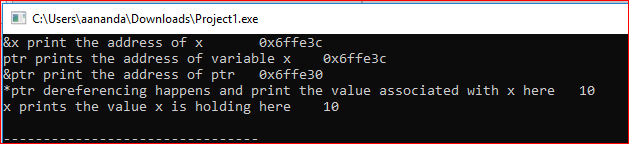Note: Now if I define dereferencing. It is a way to access or manipulate data associated with the memory location pointed by the pointer. Dereferencing can be done using * along with pointer variable name.

Now I know there is a big question in your mind why do I need a pointer and why do I need to do pointer dereferencing and other complicated tasks when I know the variable value directly. And it is easy for me to print

int x=10;
cout<<x<<endl

int x=10;
int *ptr=&x;
cout<<*ptr<<endl.

##### Why perform this cycle operation when I can directly access the value?

Yes, it is a very good question and only through this question, you can understand the need is the real importance of pointers.

##### Program on Pointers using C++:
```#include<iostream>
using namespace std;
int main()
{
int a = 10;
int *p = &a;
cout << a << endl;
cout << &a << endl;
cout << p << endl;
cout << &p << endl;
cout << *p << endl;
return 0;
}
```
###### Output: